# Tetrahedral pyramid

Determine the surface of a regular tetrahedral pyramid when its volume is V = 120 and the angle of the sidewall with the base plane is α = 42° 30´.

Result

S =  200.643

#### Solution:

$V=120 \ \\ A=42 + \dfrac{ 30 }{ 60 }=\dfrac{ 85 }{ 2 }=42.5 \ ^\circ \ \\ A_{1}=A ^\circ \rightarrow\ \text{rad}=A ^\circ \cdot \ \dfrac{ \pi }{ 180 } \ =42.5 ^\circ \cdot \ \dfrac{ 3.1415926 }{ 180 } \ =0.74176=17π/72 \ \\ \ \\ \tan A=h /(a/2) \ \\ \ \\ h=a/2 \cdot \ \tan A \ \\ V=\dfrac{ 1 }{ 3 } a^2 \ h \ \\ V=\dfrac{ 1 }{ 6 } a^3 \ \tan A \ \\ \ \\ a=\sqrt{ 6 \cdot \ V / \tan(A_{1}) }=\sqrt{ 6 \cdot \ 120 / \tan(0.7418) } \doteq 9.2277 \ \\ \ \\ h=a/2 \cdot \ \tan(A_{1})=9.2277/2 \cdot \ \tan(0.7418) \doteq 4.2278 \ \\ \sin A_{1}=h : w \ \\ \ \\ w=h / \sin(A_{1})=4.2278 / \sin(0.7418) \doteq 6.258 \ \\ \ \\ S_{1}=a \cdot \ w/2=9.2277 \cdot \ 6.258/2 \doteq 28.8733 \ \\ \ \\ S=a^2+4 \cdot \ S_{1}=9.2277^2+4 \cdot \ 28.8733 \doteq 200.6434 \doteq 200.643$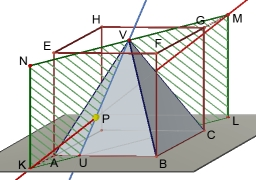Our examples were largely sent or created by pupils and students themselves. Therefore, we would be pleased if you could send us any errors you found, spelling mistakes, or rephasing the example. Thank you!

Leave us a comment of this math problem and its solution (i.e. if it is still somewhat unclear...):Be the first to comment!Tips to related online calculators
Pythagorean theorem is the base for the right triangle calculator.
Tip: Our volume units converter will help you with the conversion of volume units.

## Next similar math problems:

1. Tetrahedral pyramid 8Let’s all side edges of the tetrahedral pyramid ABCDV be equally long and its base let’s be a rectangle. Determine its volume if you know the deviations A=40° B=70° of the planes of adjacent sidewalls and the plane of the base and the height h=16 of the p
2. Wall thicknessThe hollow metal ball has an outside diameter of 40 cm. Determine the wall thickness if the weight is 25 kg and the metal density is 8.45 g/cm3.
3. Triangular prism - regularThe regular triangular prism is 7 cm high. Its base is an equilateral triangle whose height is 3 cm. Calculate the surface and volume of this prism.Calculate the volume (V) and the surface (S) of a regular quadrilateral prism whose height is 28.6 cm and the deviation of the body diagonal from the base plane is 50°.
5. Triangular prism,The regular triangular prism, whose edges are identical, has a surface of 2514 cm ^ 2 (square). Find the volume of this body in cm3 (l).
6. Triangular prismThe base of the perpendicular triangular prism is a rectangular triangle with a hypotenuse of 10 cm and one leg of 8 cm. The prism height is 75% of the perimeter of the base. Calculate the volume and surface of the prism.
7. Block or cuboidThe wall diagonals of the block have sizes of √29cm, √34cm, √13cm. Calculate the surface and volume of the block.
8. Two rectangular boxesTwo rectangular boxes with dimensions of 5 cm, 8 cm, 10 cm, and 5 cm, 12 cm, 1 dm are to be replaced by a single cube box of the same cubic volume. Calculate its surface.
9. Surface of the cubeFind the surface of the cube that has volume 1/1m3 2/0.001 m3 3/8000 mm3
10. Permille of alcohol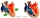I have 2 per mille of alcohol in my blood. How many milliliters is it when I have 5 liters of blood?
11. Hemisphere cutCalculate the volume of the spherical layer that remains from the hemisphere after the 3 cm section is cut. The height of the hemisphere is 10 cm.
12. KostkaKostka je vepsána do koule o poloměru r = 6 cm. Kolik procent tvoří objem kostky z objemu koule?
13. The largest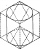The largest possible cylinder was cut from a 20 cm cube. What is the volume of this cylinder?
14. The edge of a cube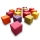How much does the edge of a cube of 54.9 cm3 measure?
15. Cuboid and ratioFind the dimensions of a cuboid having a volume of 810 cm3 if the lengths of its edges coming from the same vertex are in ratio 2: 3: 5
16. Half-filled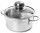A cylindrical pot with a diameter of 24 cm is half-filled with water. How many centimeters will the level rise if we add a liter of water to it?
17. Wax candles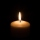A company makes wax candles in the shape of a cylinder. Each candle has a diameter of 4 inches and a height of 7 inches. If the company used 7561.12 in3 of wax, how many candles did it make? Use 3.14, and do not round your answer